## Basics

### Simple kinematics

1. Relativistic kinematics and four-momentum conservation
2. Orientation with respect to an axis (the beam axis)
3. Mandelstam variables

VARYING CENTRE-OF MASS ENERGIES

1. partons are massless;
2. they move in parallel with their parent hadron, i.e. taking the beam to be parallel to the z-axis translates into the partonic four-momentum having neither an x- nor a y-component;
3. their energy fraction w.r.t. the hadron energy is described by a quantity called the Bjorken-x (a refinement of this will be presented later), summing it over all partons inside a hadron yields one,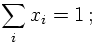4. the probability of finding a parton of a certain kind inside a given hadron is determined through a function of the Bjorken-x and the characteristic resolution scale of the partons inside a hadron, f(x,Q). It is called a parton distribution function. This function is universal for the hadron and parton species and in that sense, process-independent.
Details on this will be discussed in the QCD section.
At this point it should at least be noted that also for point-like incoming particles, such as electrons, whose energy in principle is fixed, a similar reasoning can apply. This is because charged particles are usually accompanied with a coherent cloud of virtual photons, which, as a consequence of quantum mechanics, are produced, exist for some limited time and recombine with the electron. When an interaction occurs this coherence is broken, and the electron interacts with duly reduced energy. Of course, using the same Bjorken-x idea of above, the probability of finding an electron-"parton" inside an electron peaks at x=1. Again, this will be discussed later.
In both cases, in order to calculate a cross section, the partonic initial state is not fixed any longer, implying that an integral over all configurations has to be performed. Usually this translates into the following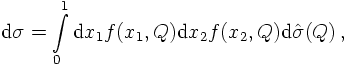where the first σ is the inclusive one, integrated over all parton configurations, and the second one is the corresponding exclusive cross section for two partons with fixed energies. It thus depends on the scale of the partonic subsystem.

CONNECTING THE BJORKEN-X WITH S AND Y

However, at this point it is worthwhile to consider the effects of this intrinsic structure of the beam particles on kinematics. According to the assumptions above, and taking all partons to be massless, their momenta can be written as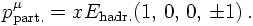Considering for simplicity symmetric collisions, where both incoming beams have the same energy, the respective centre-of mass systems are given by the hadron and the parton momenta. Denoting the hadron momenta with P, neglecting their mass and denoting the parton momenta with p, the corresponding c.m. energies squared read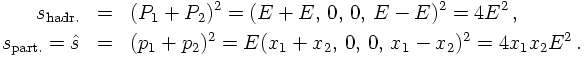Of course, the parton centre-of mass system is described by a boost along the z-axis w.r.t. the hadron c.m. system. It thus has a rapidity, given by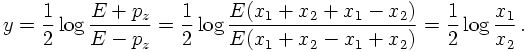Therefore, the two Bjorken-x of the two partons can be expressed through the centre-of mass energy of the partonic system and through its rapidity w.r.t. the hadron c.m. system. This connection reads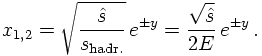SPECIFYING FOR 2→2 PROCESSES

Often, the scattering processes in question are of the type 2→ 2, see above for details. Assuming the hadronic c.m. system to be the laboratory system, the tranverse momenta of the outgoing particles just compensate each other, i.e., in two dimensions,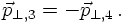Then, the absolute value of the transverse momenta and their respective rapidities in the laboratory system are connected to the Bjorken-x through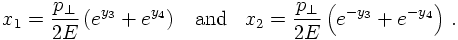This can be expressed through their average and difference,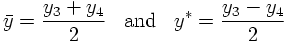as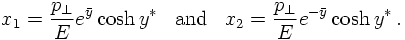In these coordinates,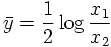and the invariant mass of the produced system reads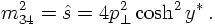5. Problems